Choose Language Hide Translation Bar
Highlighted

## DOE for Discrete Data (Categorical Type Response)

Hey JMPers!

I am struggling to make a discrete data model on JMP 12. I tried to find the answers with many of indices. Now it seems like I am stuck out.

First I thought the choice design is what I want but it probably not. The structure of data is simple. Here is an example data table. I'd like to check A and E (DF of E should be 40000 you know..)  ONLY in ANOVA table. If anybody has solutions, plz make me comfy.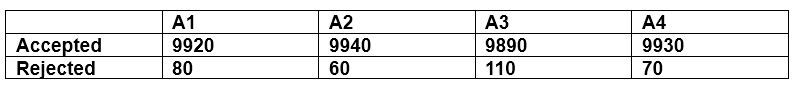1 ACCEPTED SOLUTION

Accepted Solutions
Highlighted

## Re: DOE for Discrete Data (Categorical Type Response)

The result you are showing is an Analysis of Variance which is typically shown for analysis with continuous ouputs at least for JMP anyway.  The way you originally had everything labelled it appeared that you wanted some sort of nominal regression or at least your value labels would have been recognized in JMP as nominal.  To get an answer close to what you are showing you need to convert your response to a continuous value.  For example use 1 for Accept and 2 for Reject.  These values must be continuous for you to get an Analysis of Variance report that is close to what you are showing.  You must also use the counts as Frequency when you set up your model.  The attached table has a script for the fit that you can run to recreate the model and see the Analysis of Variance report.  Another question for you, what version of JMP are you using?  The table I have attached is from JMP 13.  There are differences that would need to be described for you to run the attached scripts if you are using and older version of JMP.

I ran the model with X as a categorical and a continous factor.  For the continous model X had to added as polynomial to degree 3 to DF correct.  The answers were exactly the same either way, but both are different than what you show in your results.  So, if these results are still insufficient you will need to provide more information as to how you generated your original fit.  What software did you use originally?

8 REPLIES 8
Highlighted

## Re: DOE for Discrete Data (Categorical Type Response)

This data is categorical: your A1-A4 levels are the categorical predictor X, your Accept/Reject levels are the categorical Y, and your counts are the frequencies. Here is the JMP data table: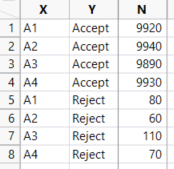Select Analyze > Fit Y by X, select X and click X, select Y and click Y, select N and click Freq. Here is the analysis: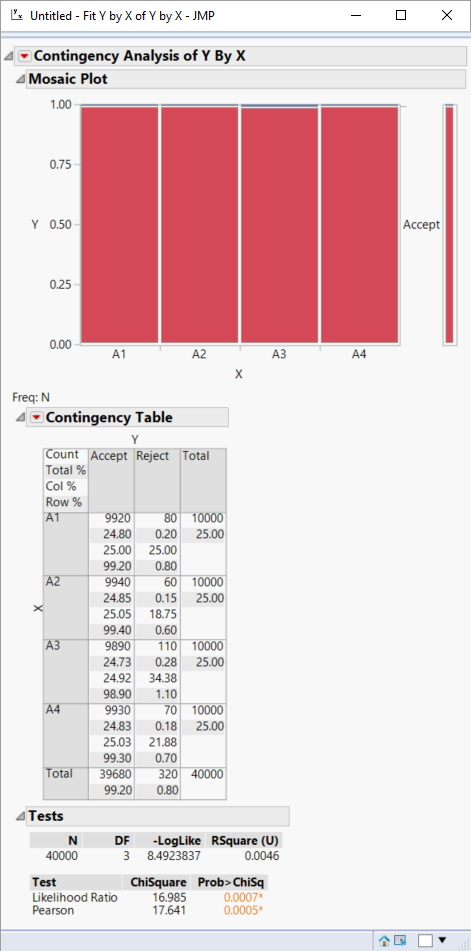You could also use logistic regression: select Analyze > Fit Model, select Y and click Y, select X and click Add, and select N and click Freq.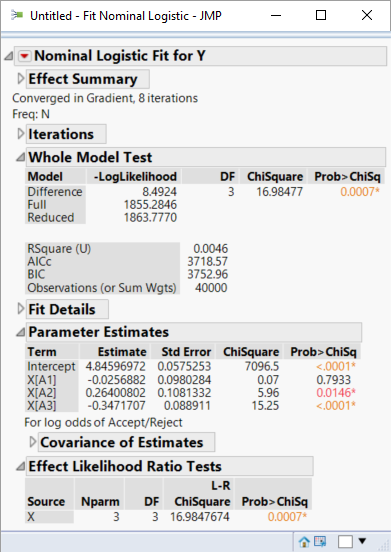The results are the same, but each platform has different options. The contingency table analysis is for a simple case of one X and one Y. The logistic regression analysis allows for more than one X and terms that are transformations of the Xs, such as X1*X2 or X1^2.

Learn it once, use it forever!
Highlighted

## Re: DOE for Discrete Data (Categorical Type Response)

Thank you for the answer but please check out what I actually meant.
Highlighted

## Re: DOE for Discrete Data (Categorical Type Response)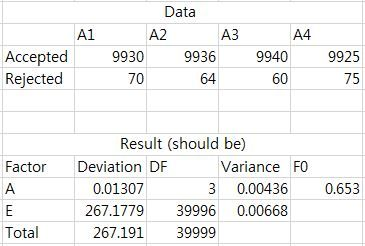I meant that I put the data above, then I want to see 'this result' on JMP result.

Highlighted

## Re: DOE for Discrete Data (Categorical Type Response)

Can you plese be more specific on A and E. It's not clear to me from the data you provided. Then we might be able to answer the missing piece, as I believe this information is within the log regression report available, just not all shown in the above screenshot.

@KrissKdash wrote:I meant that I put the data above, then I want to see 'this result' on JMP result.

Highlighted

## Re: DOE for Discrete Data (Categorical Type Response)

A is 'level' and E is 'error' and it is not a log regression report.

I just wrote down the result on spread sheet.

If I make a table with 40,000 rows (4 levels, 10,000 repetitions each), then I can see the result below.

However, I would like to make a table as simple as possbile like,,

1 9930

1 70

2 9936

2 64

3 9940

3 60

4 9925

4 75

and like to check the same result beblow.

Can you show me how to do it?

Highlighted

## Re: DOE for Discrete Data (Categorical Type Response)

The result you are showing is an Analysis of Variance which is typically shown for analysis with continuous ouputs at least for JMP anyway.  The way you originally had everything labelled it appeared that you wanted some sort of nominal regression or at least your value labels would have been recognized in JMP as nominal.  To get an answer close to what you are showing you need to convert your response to a continuous value.  For example use 1 for Accept and 2 for Reject.  These values must be continuous for you to get an Analysis of Variance report that is close to what you are showing.  You must also use the counts as Frequency when you set up your model.  The attached table has a script for the fit that you can run to recreate the model and see the Analysis of Variance report.  Another question for you, what version of JMP are you using?  The table I have attached is from JMP 13.  There are differences that would need to be described for you to run the attached scripts if you are using and older version of JMP.

I ran the model with X as a categorical and a continous factor.  For the continous model X had to added as polynomial to degree 3 to DF correct.  The answers were exactly the same either way, but both are different than what you show in your results.  So, if these results are still insufficient you will need to provide more information as to how you generated your original fit.  What software did you use originally?

Highlighted

## Re: DOE for Discrete Data (Categorical Type Response)

Missed where you were using JMP 12. Right click on the red triangle next to the Fit Least Squares script and select Run.
Highlighted

## Re: DOE for Discrete Data (Categorical Type Response)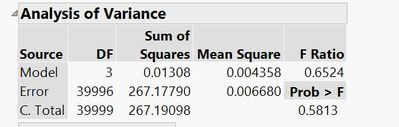For the analysis in the attached table I used your original values you gave at the beginning of this string.  I went back and input your later values and got the Analysis of Variance shown below.

Article Labels

There are no labels assigned to this post.